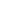# Exercise Your Brain with Amusing Mathematical Problems

Amusing mathematics stimulates logical and creative thinking in order to find solutions of particular problems and discover the beauty of mathematics. During the classes, the students will work independently and in small groups with the support of the teacher on solving a variety of recreational mathematical problems. These problems are known for centuries introduced in Liber Abaci (1202) by Leonardo of Pisa known as Fibonacci; Problems to Sharpen the Young (circa. 9th century) by Alcuin, and A Tangled Tale by Lewis Carroll. Solving a problem of amusing mathematics brings genuine creative joy of finding the truth and beauty of science.

#### DELIVERY MODE

• Face-to-Face

• L.E. Sigler. Fibonacci's Liber Abaci. Springer-Verlag, 2002.
• Lewis Carroll: The Mathematical Recreations of Lewis Carroll: Pillow Problems and a Tangled Tale. Dover: Courier, 1958.
• M. Gardner. Mathematical Puzzles and Diversions. London: Bell and Sons, 1961.

#### COURSE OUTLINE

• Introduction to recreational mathematics. “Brain stretching” by solving simple though not-evident problems and paradoxes. Working in pairs and small groups to find solutions of 12 recreational mathematical problems.
• Discussion of found solutions with the class. Appearance of these problems in mathematical textbooks of different times, when relevant. More problems on the same mathematical methods to work on independently.
\$153 Limited / \$138

<p>Amusing mathematics stimulates logical and creative thinking in order to find solutions of particular problems and discover the beauty of mathematics. During the classes, the students will work

...
12 Nov
Mo Tu We Th Fr Sa SuInterested in this course but can't attend? Please join the waiting list by clicking .